# Nitrogen tribromide (NBr3) lewis dot structure, molecular geometry, polar or non-polar, hybridization

Home  > Chemistry Article > NBr3 lewis structure and its molecular geometry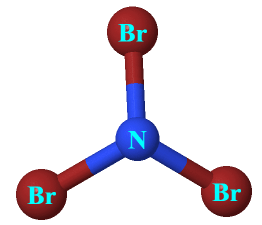Nitrogen tribromide appears as deep red is a chemical compound having the molecular formula NBr3. In pure form, it is very explosive in nature. It is highly unstable and can be hydrolyzed in water.

In this article, we will discuss NBr3 lewis structure, molecular geometry, polarity, bond angle, hybridization, etc.

Properties of Nitrogen tribromide

• It explodes at -100 °C.
• It is a very unstable and explosive chemical compound.
• It appears as a deep-red and volatile solid.
• It has a molar mass of 253.7 g/mol.
 Name of Molecule Nitrogen tribromide Chemical formula NBr3 Molecular geometry of NBr3 Trigonal pyramid Electron geometry of NBr3 Tetrahedral Hybridization Sp³ Bond angle <109.5º Total Valence electron for NBr3 26 The formal charge of NBr3 0
Page Contents

## How to draw lewis structure for NBr3

NBr3 lewis structure contains three N-Br bonds, nitrogen in center position whereas all three bromine atoms are at the terminal position. There is only one lone pair present on the central atom in the NBr3 lewis structure.

Each bromine atom at the surrounding position contains three lone pairs and is connected to the central atom with a single bond.

Let’s see how to draw the lewis structure of NBr3 with a good approach.

## Follow some steps for drawing the lewis structure of NBr3

1. Count total valence electron in NBr3

Valence electrons are usually the electrons found in the outermost shell of an atom. To know the total valence electron in the NBr3 molecule, we get to know the valence electron of an individual atom(nitrogen and bromine).

The nitrogen atom belongs to the 15th or 5A group in the periodic table, therefore, the electrons present in its outer shell are 5 whereas the bromine atom is a member of the halogen family and has 7 electrons in its outer shell.

⇒ Total valence electron in Nitrogen = 5

⇒ Total valence electron in Bromine = 7

∴ Total valence electron available for drawing the NBr3 lewis structure = 5 + 7*3 = 26 electrons     [∴NBr3 contains one nitrogen and three bromine atoms]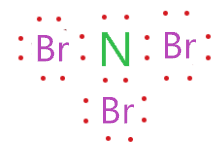2. Find the least electronegative atom and placed it at center

The atom with less electronegativity is more prone to share the electrons than the atom with higher electronegativity. The electronegativity of nitrogen and bromine atom is very near to each other, so, the other method to determine the central atom of the lewis diagram is by observing the least numerous element in the compound.

The element which repeats least in the compound should be the central atom in the lewis diagram. So, in the case of NBr3, the nitrogen atom repeated only one time whereas the bromine atom repeats three times.

Hence, put the nitrogen atom at central position whereas spread the three bromine atom around it.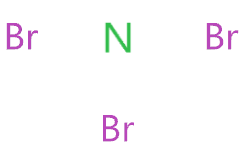3. Connect nitrogen and bromine atoms with a single bond

Here, we will join together each bromine atom to the nitrogen central atom via a single bond.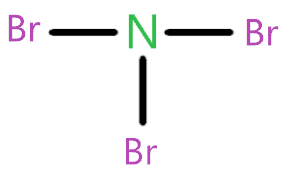Now we need to know how many valence electrons we used in the above structure before moving to the next step. As we see in the above structure, there is three single bond used and one single bond made up of two valence electrons, hence, (3×2) = 6 valence electrons are used in the above structure from a total of 26 valence electrons for NBr3 lewis structure.

∴ (26 – 6) = 20 valence electrons

Now we are left with 20 valence electrons more.

4. Placed remaining valence electrons starting from outer atom first

The outer atom in the NBr3 molecule is bromine that needs 8 electrons to complete its octet and attains stability. Let’s try to put the remaining valence electrons around each bromine atom till they complete their octet.By putting 6 valence electrons represented as dots around each bromine atom in the above structure, we see that they completed their octet comfortably as all three bromine atoms have 8 electrons(6 electrons represent as dots + 2 electrons in forms of single bond) in its octet shell.

Now, we have only two valence electrons left as in the above structure, we use (three single bonds that contains 6 electrons + 18 electrons as dots)

5. Complete central atom octet  and use covalent bond if necessary

So, we still have 2 valence electrons in our pocket, and nitrogen as a central atom also needs 8 electrons to complete its octet but it already sharing 6 electrons through the three single bonds connected to the bromine atoms.

Hence, nitrogen needs only 2 electrons to complete its complete, therefore, put the 2 remaining valence electrons on it.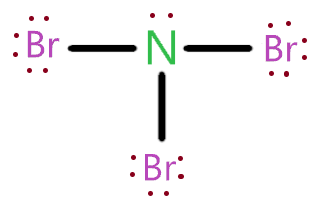### NBr3 lewis structure

So, in the above structure, each atom(bromine and nitrogen) completed its octet and we also used all the valence electrons that are available for drawing the lewis structure of NBr3.

## What are the electron and molecular geometry of NBr3?

The molecular geometry of NBr3 is trigonal pyramidal, and electron geometry is tetrahedral because the lone pair present on the central atom creates repulsion between adjacent bonded pairs of electrons, as a result, two bromine atom in equatorial position pushes far apart giving its molecular geometry same as a trigonal pyramid.### NBr3 molecular geometry

The generic formula for NBr3 is AX3N1. So, according to the VSEPR chart, if the molecule has the formula of AX3N1  then the molecule shape of that molecule is trigonal pyramidal, and electron geometry is tetrahedral.

 Bonded atoms Lone pair Generic formula Hybridization Molecular geometry Electron geometry 1 0 AX S Linear Linear 2 0 AX2 Sp Linear Linear 1 1 AXN Sp Linear Linear 3 0 AX3 Sp² Trigonal planar Trigonal planar 2 1 AX2N Sp² Bent Trigonal planar 1 2 AXN2 Sp² Linear Trigonal planar 4 0 AX4 Sp³ Tetrahedral Tetrahedral 3 1 AX3N1 Sp³ Trigonal pyramid Tetrahedral 2 2 AX2N2 Sp³ Bent Tetrahedral 1 3 AXN3 Sp³ Linear Tetrahedral 3 2 AX3N2 Sp³d T-shaped Trigonal bipyramidal

## Hybridization of NBr3

The hybridization of NBr3 can easily find out by knowing the steric number of its central atom.

The steric number is an addition of bonded atoms to the central atom and the presence of lone pair on that central atom.

∴ Steric number of NBr3 = (Number of bonded atoms attached to nitrogen + Lone pair on nitrogen)

As we see in the lewis structure of NBr3, there are three bromine atoms bonded to the nitrogen central atom and only one lone pair present on the nitrogen central atom.

∴ Steric number of NBr3 = (3 + 1) = 4

The steric number of 4 implies the hybridization state in the NBr3 molecule is Sp3.

## The bond angle of NBr3

“The ideal bond angle for the Nitrogen tribromide is 109.5° since it has a Trigonal pyramidal molecular geometry. Experimentally we would expect the bond angle to be approximately Less than 109.5°.”[check here]

## Nitrogen tribromide polarity: is NBr3 polar or nonpolar?

NBr3 is a polar molecule because the one lone pair present on the nitrogen central atom generates repulsion between electrons pairs that makes the overall structure bent and this creates unequal charge distribution of charge within the structure that generates a permanent dipole moment.

Let’s understand it with the help of three factors. (a). Electronegativity (b). Dipole moment (c). Molecular shape

## Three factors that indicate the polarity of NBr3

1. Electronegativity:

Electronegativity means the tendency of an atom to attracting electrons towards itself. If the electronegativity difference between the atoms is high then the polarity will also be higher. Now, look at the electronegativity of nitrogen and bromine.

The difference between the electronegativity of nitrogen and bromine is directly proportional to the Polarity of the NBr3 molecule.

The electronegativity of nitrogen is 3.04 and for bromine, it is 2.96. And the difference of electronegativity between nitrogen and bromine is 0.08 which is very little.

So, the N-Br bond is non-polar due to less electronegativity difference among atoms but the overall molecule is polar due to the presence of lone pair on the central atom of the NBr3.

2. Dipole moment

Dipole moment ensures the strength of polarity between nitrogen and bromine atoms. As greater the dipole moment of the molecule, the more is the polar nature of that molecule.

The dipole moment of NBr3 is very less but still, it is non-zero that causes NBr3 to become polar in nature.

In mathematical terms, dipole moment can be expressed as-

⇒ Dipole moment formula =  charge on the atoms * the distance between them

∴ D = Q × R

3. Geometrical or molecular shape

The geometrical shape is also a big factor to determine is NBr3 polar or non-polar.

As we know the molecular shape of NBr3 is trigonal pyramidal which is asymmetric and when the shape of the molecule is asymmetric or distorted it doesn’t cancel the dipole moment across the molecule. Hence cause polar nature of the molecule

All these factors are enough to understand why NBr3 is polar in nature.

## FAQ

### How many bond pair and lone pair a central atom has in NBr3 lewis structure?

In the NBr3 lewis structure, only one lone pair is present on the nitrogen central atom whereas three bonded pairs are attached to the outer atom(bromine).

### Why NBr3 molecular geometry is the trigonal pyramid and its electron geometry is tetrahedral?

Always remember, molecular geometry only considers bond pairs of electrons to determining the shape of any molecule, and electron geometry considers both bond pair and lone pair to determine the geometry of any molecule.

As NBr3 contains one lone pair and three bonded pairs. So, according to the VSEPR chart, the electron geometry of NBr3 is tetrahedral while its molecular geometry is trigonal pyramidal.

### Is the lewis structure of NBr3 the same as NF3 and NCl3?

Yes, the lewis structure of NBr3 is almost the same as NF3 and NCl3.

NF3 lewis structure has 3 fluorine and 1 nitrogen atom connected with three single bonds and NCl3 lewis structure has 3 chlorine and 1 nitrogen connected with three single bonds also.

In all these molecules(NBr3, NF3, and NCl3), there is one lone pair present on the central atom.

## Summary

Now it’s time to take a quick review of an overall article with the help of some bulleted points.

• The total valence electron available for the NBr3 lewis dot structure is 26.
• The hybridization of NBr3 is Sp³.
• Nitrogen tribromide is slightly polar in nature.
• The molecular geometry of NBr3 is trigonal pyramidal and its electron geometry is tetrahedral.
• Lewis structure of NBr3 contains 1 lone pair and 3 bonded pairs.
##### Subscribe to Blog via Email

Join 2 other subscribers

Share it...